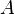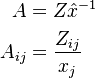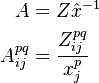# Technical Coefficient Matrix

## Definition

A Technical Coefficient Matrix (also known as the Technology Matrix, Direct Coefficients or Direct Requirements Matrix) is the collection of input-output coefficients .

This matrix is a central element of an Input-Output Model. It is derived by dividing the Input-Output Matrix (Z) by the vector of Total Output (x), thereby generating a normalized representation of economic structure that is independent of the units used to quantify exchanges (whether those are monetary or physical units).

The input coefficients for all of the intermediate inputs and all value added components add up to one (100%).

## Formula

The technical coefficients matrix is often in IO literature denoted as the$A$ matrix and it is given by the multiplication of Z with the diagonalised and inverted industry output x:\begin{align} A & = Z\hat{x}^{-1} \\ A_{ij} & = \frac{Z_{ij}}{x_j} \end{align}

In the case of a Multiregional Input-Output Model the generalized expression is:\begin{align} A & = Z\hat{x}^{-1} \\ A^{pq}_{ij} & = \frac{Z^{pq}_{ij}}{x^{p}_j} \end{align}

## Issues and Challenges

• “Accounting coefficient” would be a better term than “technical coefficient”, since what we have are monetary amounts and not quantities. However, the term “technical coefficient” is generally used.